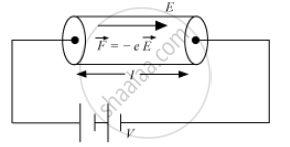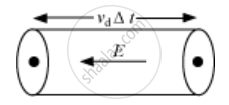# Using the Concept of Free Electrons in a Conductor, Derive the Expression for the Conductivity of a Wire in Terms of Number Density and Relaxation Time. Hence Obtain the Relation Between Current Density and the Applied Electric Field E. - Physics

Using the concept of free electrons in a conductor, derive the expression for the conductivity of a wire in terms of number density and relaxation time. Hence obtain the relation between current density and the applied electric field E.

#### SolutionThe drift velocity of electrons in a conductor is given as

V_d = (eEr)/m  ...(1)

where,
E = electric field set up across a conductor
m = mass of the electron
τ" data-mce-style="display: inline; font-style: normal; font-weight: 400; line-height: normal; font-size: 16px; text-indent: 0px; text-align: left; text-transform: none; letter-spacing: normal; word-spacing: 0px; word-wrap: normal; white-space: nowrap; float: none; direction: ltr; max-width: none; max-height: none; min-width: 0px; min-height: 0px; border: 0px; padding: 0px; margin: 0px; color: #212121; font-family: Roboto, sans-serif; font-variant-ligatures: normal; font-variant-caps: normal; orphans: 2; widows: 2; -webkit-text-stroke-width: 0px; background-color: #ffffff; text-decoration-style: initial; text-decoration-color: initial; position: relative;" data-mce-tabindex="0">ττ = average relaxation time
Now the current flowing in the conductor can be derived asElectron drift to a small distance in a time Δt = VdΔt

Amount of charge passing through the area A in time Δt, q = IΔt

IΔt = neAvdΔt

or I = neAvd   ...(2)

Where,

→ Number of free electrons per unit volume or number density.

Now from equation (1) and (2), we get

I = ("ne"^2ArE)/m ....3

Since the resistivity of a conductor is given as

rho = m/("ne"^2r)

Now, we know that conductivity of a conductor is mathematically defined as the reciprocal of resistivity of the conductor. Thus

rho = 1/sigma ....(4)

where sigma = conductivity of the conductor. Thus, from equation (3) and (4), we get

sigma = ("ne"^2r)/m ...(5)

Now, from equation (3) and (5), we have

I/A = sigmaE .....(6)

and the current  density is given as

J = I/A

Thus J =sigmaE

Concept: Electrical Resistivity and Conductivity
Is there an error in this question or solution?